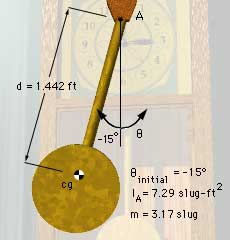Ch 6. Rigid Body Force and Acceleration Multimedia Engineering Dynamics Moment of Inertia Translational Motion Fixed Point Rotation GeneralPlane Motion
 Chapter - Particle - 1. General Motion 2. Force & Accel. 3. Energy 4. Momentum - Rigid Body - 5. General Motion 6. Force & Accel. 7. Energy 8. Momentum 9. 3-D Motion 10. Vibrations Appendix Basic Math Units Basic Equations Sections Search eBooks Dynamics Fluids Math Mechanics Statics Thermodynamics Author(s): Kurt Gramoll ©Kurt GramollDYNAMICS - CASE STUDY IntroductionProblem Graphic The pendulum for the grandfather clock in the previous Moment of Inertia section rotates about a pin through the top of the pendulum. In order to build clocks that don't collapse, the engineers need to determine the force that the pin exerts on the pendulum as it swings. What is known: The pendulum is released from rest at an angle of 15° to the left of vertical. The distance from the top of the pendulum to its center of gravity is d = 1.442 ft. The pendulum has a moment of inertia about the top IA = 7.289 slug-ft2 (calculated in previous section). The pendulum has a mass m = 3.17 slugs.Problem Diagram Question When the pendulum reaches a vertical position, what is the angular acceleration of the pendulum? What force is exerted on the pendulum by the pin? Approach Use the relationship between applied moment and moment of inertia to determine the angular acceleration. Use Newton's Second Law to determine the unknown forces acting on the pendulum.

Practice Homework and Test problems now available in the 'Eng Dynamics' mobile app
Includes over 400 problems with complete detailed solutions.
Available now at the Google Play Store and Apple App Store.